# Fundamentals of Electric Power Measurements

### Basic Electrical Power Measurements

Understanding power generation, power loss, and the different types of power measured can be intimidating. Below is an overview of basic electric and mechanical power measurements.

#### Electric Current, Voltage, and Resistance

Any discussion of electricity inevitably leads to electric current, voltage, and resistance. These concepts are shown below in Figure 1. Electric current is the flow of electricity itself and is measured in units called amperes (A). Voltage is the force that compels electricity to flow and is measured in units called volts (V or U). Resistance expresses the difficulty with which electricity flows and is measured in units called ohms (Ω).

The figure below shows these relationships as electric circuits. In an electric circuit, electric current passes through various load types that include resistance, inductance and capacitance from positive polarities on power sources such as batteries, and then returns to negative polarities on the power source. Load is the term generally used to refer to something that acquires electricity from a power source and does work (provide light, in the case of a light bulb).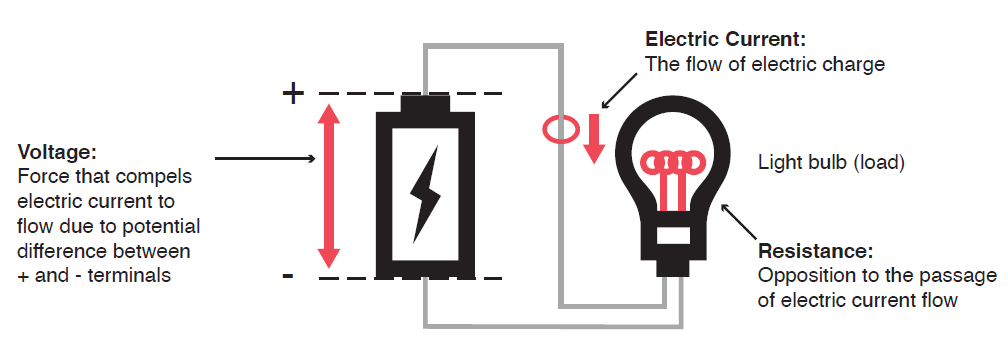Figure 1 - Basic components of electrical circuit

#### Power

Electrical energy can be converted into other forms of energy and used. For example, it can be converted into the heat in an electric heater, the torque in a motor, or the light in a fluorescent or mercury lamp. In these kinds of examples, the work that electricity performs in a given period (or the electrical energy expended) is referred to as electric power. The unit of electric power is watt (W). 1 watt is equivalent to 1 joule of work performed in 1 second.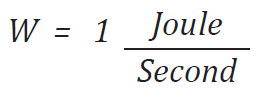In electrical systems, voltage is the force required to move electrons. Current is the rate of the flow of charge per second through a material to which a specific voltage is applied. By taking the voltage and multiplying it by the associated current, the power can be determined.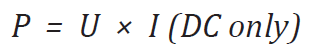#### Direct Current (DC) Power

Direct current, or DC, refers to power systems that use one polarity of voltage and current, however amplitude may vary (cyclic or random).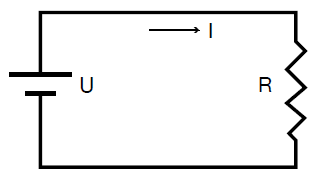Figure 2 - Basic circuit showing voltage and current with a DC voltage source
##### Ohm’s Law

A number of formulas are used with electric circuit calculations, but it is Ohm’s Law that shows the most fundamental relationship: that between electric current, voltage, and resistance. Ohm’s law states that electric current flows proportionally to voltage. The following shows a formula for expressing the relationship between current (I) and voltage (U).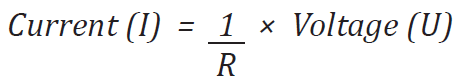Under this formula, current (I) decreases as the R value increases and, conversely, current (I) increases as the R value decreases. R here represents resistance (or electric resistance). In other words, we see that as resistance (R) increases or decreases, current flows with lesser or greater ease. This formula can be rewritten as shown below. If two values from among current, voltage, and resistance are known, you can obtain the remaining value.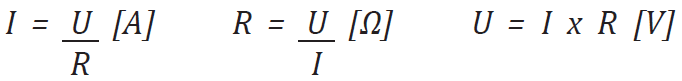The direct current (DC) power P (W) is determined by multiplying the applied voltage (U) by the current I (A), as shown above. In the example below, the amount of electrical energy determined by the previous equation is retrieved from the power supply and consumed by resistance R (in ohms) every second. Under Ohm’s Law, we can rewrite the formula as follows: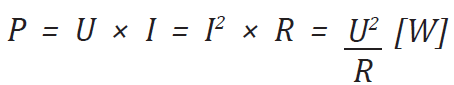Direct-current electrical circuits maintain a constant current and voltage, with no cyclic changes in either. It is thus a simple matter to obtain direct current power (P) with the resulting waveform below.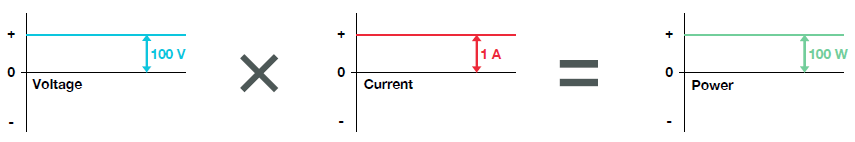#### Alternating Current (AC) Power

The power normally used in Japan is operated at 100V AC. This 100 V is voltage expressed as the root mean square (rms).

The 100 V from the wall sockets is observed as clean sine waves, as shown in the figure below. We can see that polarity changes in cycles, and that voltages are constantly fluctuating. AC voltage waveforms have clean sine waves like the graph in Figure 3, as well as a variety of other waves such as distorted waves, like common shapes as a triangular and square wave. To ascertain the size of these alternating current and voltage waves, we need values that use the same standard. Therefore the root mean square (rms), which was established based on direct current and voltage, is used.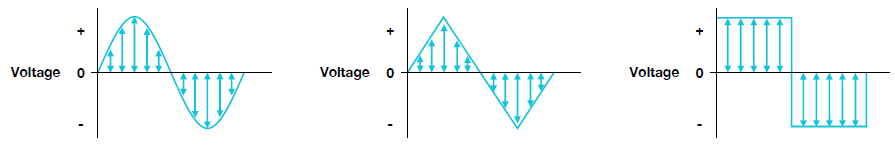Figure 3 - AC Voltage polarity changes in cycles in sine, triangular, and square waves
##### Root Mean Square (rms)

Root mean square is most commonly used when expressing the values of alternating current and voltage, and is measured in Arms and Urms. In the example above, 100 V is voltage expressed as the root mean square (rms).

The simple average of a sine wave is zero, so a different equation is required. This is why the root mean square (rms), which was established based on direct current and voltage, is used. It is based on the amount of work done by a certain amount of direct current and voltage and expresses--using the same values as the direct current and voltage--the size of alternating current and voltage that does the same amount of work.

If the calorific value when direct current voltage is applied to a resistor is the same as the calorific value when alternating current of a different waveform is applied, that alternating current voltage’s rms is the same value as for the DC voltage.

For example, the calorific value when direct current voltage of 100 V is applied to a 10 Ω resistor is the same as the calorific value when alternating current of 100 V is applied to the same resistor. The rms concept is the same for electric current.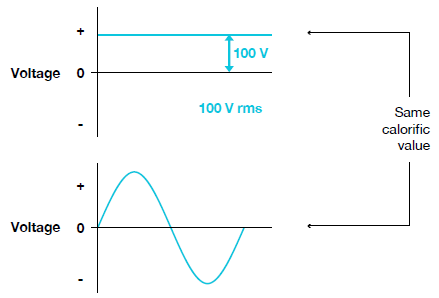Figure 4 - Equal calorific values between DC and AC signals

Calorific value refers to the amount of work done, so the following formula calculates power as a calorific value.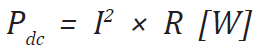As an example, the following diagram shows power fluctuation according to time when direct current of 1 A and alternating current of 1 Arms are applied to a 10 Ω resistor.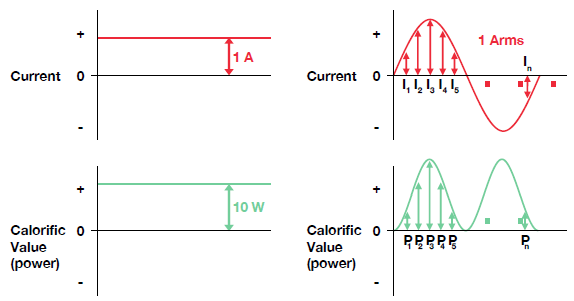Figure 5 - Power versus time with DC and AC current

Because there is no fluctuation in current value with direct current, the power value remains a constant 10 W. However, because current value constantly fluctuates with alternating current, the power value fluctuates along with time. That these two types of power (calorific values) are equal is the same as saying that the averages of Pdc and P1 – Pn are equal. This is expressed as a formula below.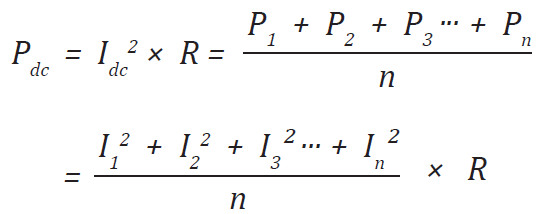Here, the resistor (R) is constant so it can be ignored. The following expresses the resulting relationship between direct current and alternating current.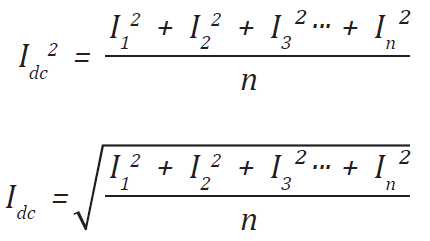Making the interval between I1 and In as small as possible in this formula, ultimately Irms gives the square root of the area of the portion enclosed in the waveform, divided by time. This is expressed as a formula below.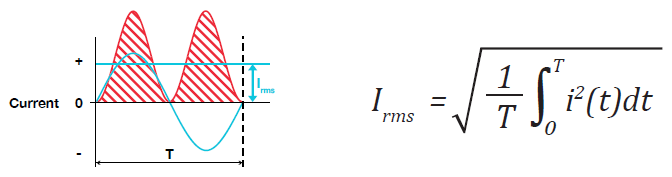The important thing to know is that direct current of 1 A performs the same amount of work as alternating rms current of 1 Arms. With constant and steady state direct current, you can obtain the power value simply by multiplying current by voltage.

Alternating current, however, is not as simple as direct current because of the phase difference between current and voltage. There are the below three types of AC power. Generally, power and power consumption refer to active power.

### Power in AC Systems

As with direct current, the power value (instantaneous power value) at a certain point in time for alternating current can be obtained by multiplying voltage and current for that point in time.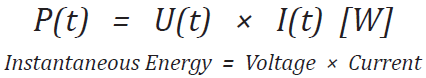With alternating current, because both current and voltage fluctuate in cycles, power values also constantly fluctuate. This is shown by the following diagram.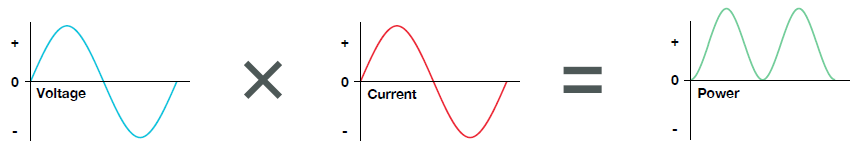As energy per second, power can be obtained from the average of the instantaneous energy, i.e., the area of the portion enclosed in the waveform, by time. The formula is as follows: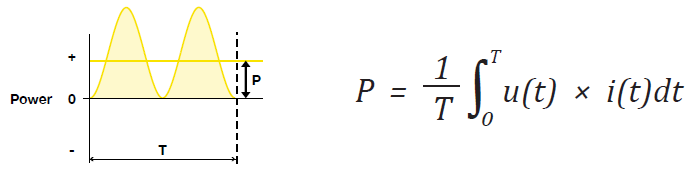For example, if a current of 1 Arms and voltage of 100 Urms is applied to a resistor, as shown below, power becomes 100 W when calculated using the formula above.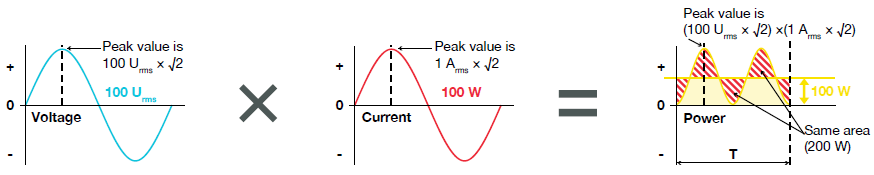When applying current and voltage to a resistor, the resulting waveforms are as shown in Figure 6 below.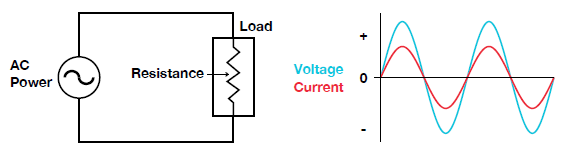Figure 6 - No phase difference in a purely resistive load

Current and voltage are said to be “in phase” in polarity and time when current and voltage waveforms pass zero. Current and voltage are always in phase when load comprises only resistance.

When a load has a coil in addition to resistance, a phase shift between the voltage and current signal occurs. This lag is called phase difference, shown in Figure 7.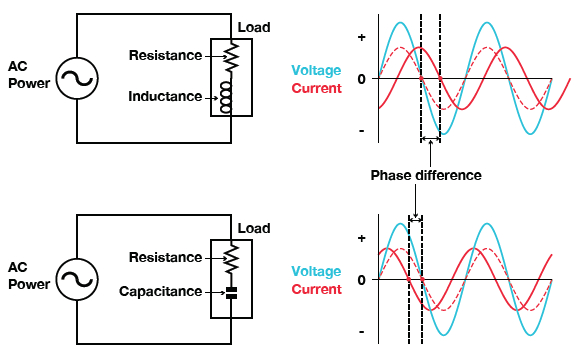Figure 7 - Phase differences representative of an inductive and capacitive load

Phase difference is generally expressed as Φ (phi) and the unit is radians but is often shown in degrees. In the example below, point A starts from point P and makes one revolution around the circumference of circle O. The distance between point A and the straight line that goes through center O and point P (red line) as the Y axis and AOP (φ) as the X axis results in the sine wave below.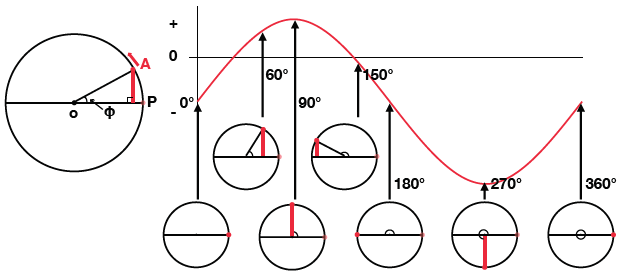Figure 8 - Sine wave illustrated with phase

Figure 9 shows a current and voltage waveform out of phase by 60°. In considering the position on the circumference of voltage (u) and current (i) as per the example above, uoi is constant at every point in time. The angle of this uoi indicates the size of the phase difference between voltage (u) and current (i).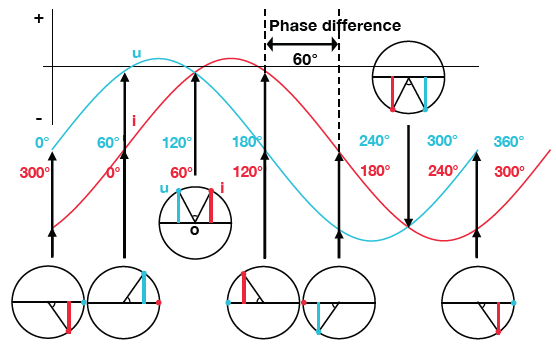Figure 9 - Voltage and current sine waves with phase difference

The three types of AC circuit load are shown in Figure 10. As shown below, phase differences between current and voltage occur based on load type.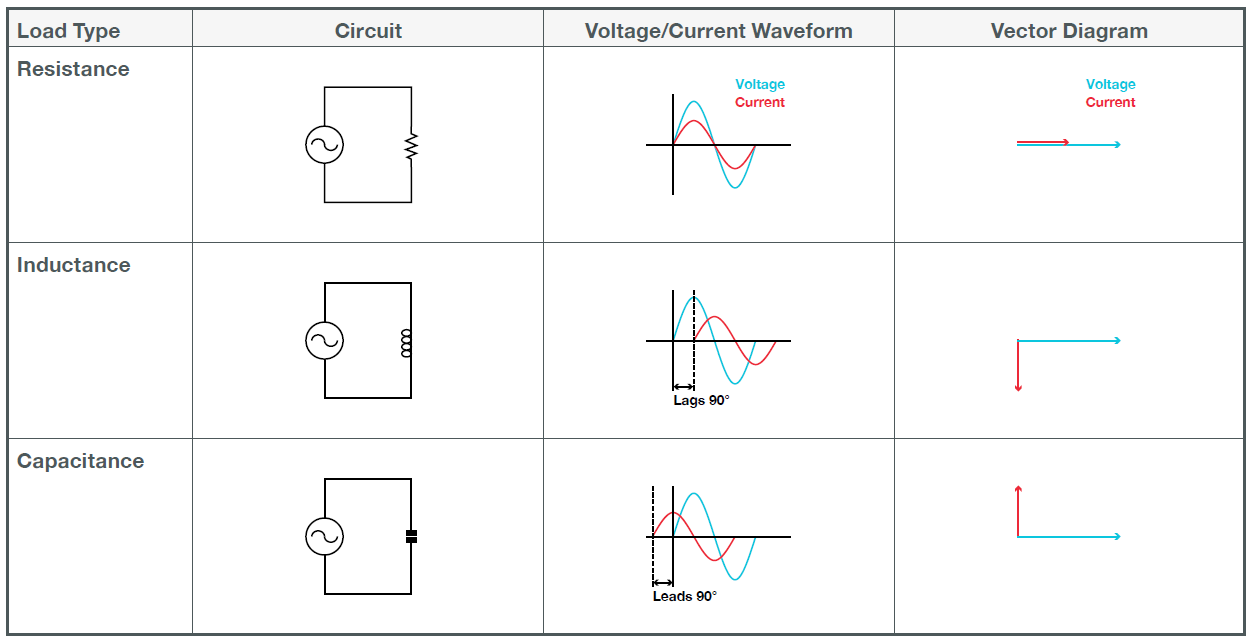Figure 10 - Phase and vector representation of AC circuits with resistive, inductive, or capacitive load

With phases, current can lag in relation to voltage or it can lead. Current lags by 90⁰ when load comprises only inductance and leads by 90⁰ when only capacitance. When all three types exist, phase difference fluctuates according to the ratio of the sizes of each component. Next, let us look at power when there is a phase difference between current and voltage.

##### AC Power with Phase Difference

When there is a phase difference between current and voltage, instantaneous energy change occurs as shown in Figure 11.

When either current or voltage is 0, instantaneous power becomes 0. As current and voltage polarities reverse in the spaces in-between, instantaneous power becomes negative. Power is the average of instantaneous energy, so power becomes smaller than it does when current and voltage are in phase (dotted line).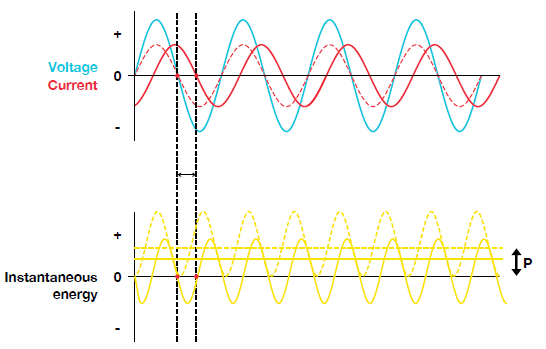Figure 11 - Instantaneous energy when voltage and current have phase difference

#### Power Triangle and Power Factor

AC circuits containing capacitance, inductance, or both contain real power and reactive power. The power triangle shown in Figure 12 helps illustrate power consumption in an inductive or capacitive circuit. The power triangle is a representation of a right triangle showing the relation of 4 main elements, active power, reactive power, apparent power, and power factor.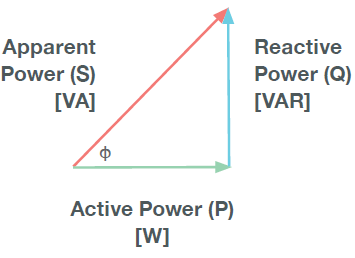Figure 12 - Power triangle shows relationship of real and reactive power.

##### Active Power

Active power (P) is the true power that a device consumes and performs the real work within an electrical circuit. Active power is calculated below with the units of watts (W).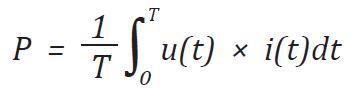##### Reactive Power

Reactive power (Q) is the power that is not consumed by the device and goes back and forth between the power supply and the load. Sometimes called wattless power, reactive power takes power away from the circuit due to the phase shift created by capacitive and/or inductive components. This phase shift reduces the amount of active power to perform the work and complicates the power calculation. Reactive power is calculated below and has the units of volt-amperes reactive (VAr). There is no reactive power in a DC circuit.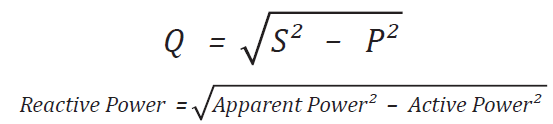##### Apparent Power

Apparent power (S) is the hypotenuse of the power triangle, consisting of the vector addition of the active power (P) and reactive power (Q). The calculation for apparent power is the rms voltage multiplied by the rms current with units of volt-amps (VA).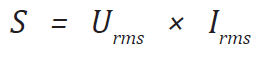##### Power Factor

In determining the power factor for sine waves, the power factor is equal to the cosine of the angle between the voltage and current (Cos Φ). This is defined as the "displacement" power factor and is correct for sine waves only. For all other waveforms (non-sine waves), the power factor is defined as power in watt divided by apparent power in voltage-amperes. This is called the "true" power factor and can be used for all waveforms, both sinusoidal and non-sinusoidal, using the qualifier λ (lambda).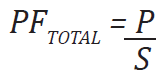The power factor (λ) increases or decreases based on the size of the phase difference (φ). Figure 13 illustrates this phenomenon.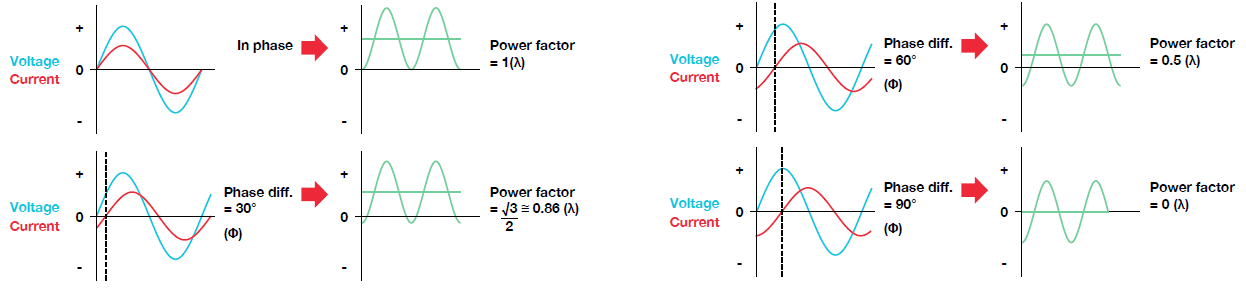Figure 13 - Power factor with various phase differences

For ideal sine waves, the current and voltage are in phase, apparent power and active power become equal, and the power factor is 1. The power factor decreases as the phase difference increases; the power factor is 0.5 (active power is 1/2 of apparent power) at a phase difference of 60⁰, and 0 at a phase difference of 90⁰. A power factor of 0 means that current is flowing to a load but it is not doing any work.

#### Vector Display of Alternating Current

The time offset between the voltage and current is called the phase difference, and Φ is the phase angle. The time offset is mainly caused by the load that the power is supplied to. In general, the phase difference is zero when the load is purely resistive. The current lags the voltage when the load is inductive. The current leads the voltage when the load is capacitive.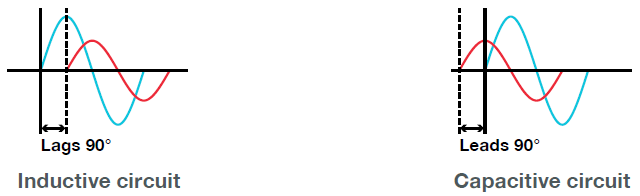Figure 14 - Phase offset between voltage and current with a purely inductive or capacitive load

A vector display is used to clearly convey the magnitude and phase relationships between the voltage and current. A positive phase angle is represented by a counterclockwise angle with respect to the vertical axis.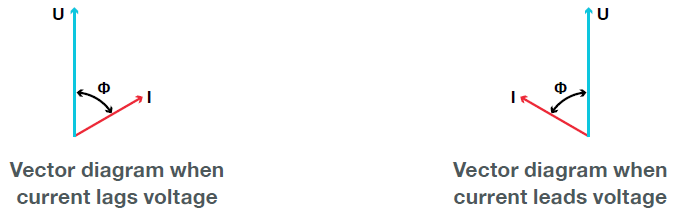Figure 15 - Vector diagram conveys magnitude and phase relationships between voltage and current

### AC Power Systems

AC power may come in single phase or polyphase formats. Single-phase electricity is used to supply common domestic and office electrical appliances, but for distribution of electrical power and to supply electricity directly to higher power equipment, three- phase AC systems are almost universally used.

#### Single-phase Wiring Diagrams

There are two common wiring configurations for single phase circuits. The most common is the single-phase, two-wire circuit. The other is the single-phase three-wire circuit, commonly found in household appliances.

#### Single-phase 2-wire system (1P2W)

This supplies single-phase AC power using two lead conductors. The simplest system, it is used when connecting power supplies to many electrical devices such as consumer electronics. When wiring a wattmeter to a single-phase, two-wire system, there are a few things to consider before wiring.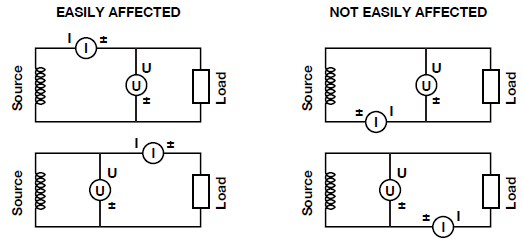Figure 16 - Various wiring diagrams of a single-phase, two-wire system

##### Effects of Stray Capacitance

When measuring a single-phase device, the effects of stray capacitance on measurement accuracy can be minimized by connecting the instrument’s current input terminal to the side that is closest to the earth potential of the power supply.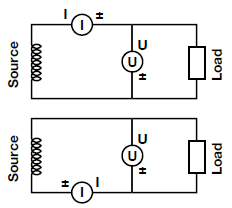Figure 17 - Wiring diagram to minimize stray capacitance

##### Effects of the Measured Voltage and Current Amplitudes

When the measured current is relatively large, connect the voltage measurement terminal between the current measurement terminal and the load. When the measured current is relatively small, connect the current measurement terminal between the voltage measurement terminal and the load.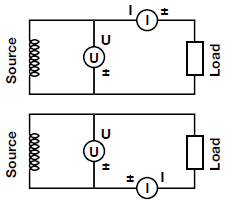Figure 18 - Wiring diagram when measured current is relatively large

#### Split-Phase 3-Wire System (1P3W)

This supplies single-phase AC power using three lead conductors. The single-phase 3-wire system is the most common power distribution system. The electricity supplied to most households is supplied using this system. The following requires two wattmeters to measure the two voltages (U1, U2) and two currents (I1, I2).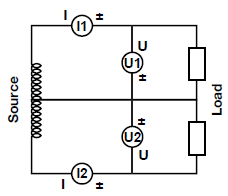Figure 19 - Split-phase 3-wire system

#### Three-phase Wiring Diagrams

Unlike single-phase systems, the conducting wires in a three-phase power supply each carry an alternating current of the same frequency and voltage amplitude relative to a common reference but with a phase difference of one third the period. Three phase systems have advantages over single-phase that make it suitable for transporting power and in applications such as induction motors.

##### Characteristics of Three-Phase Systems
• The current through and voltage across each phase has a phase difference of 120° in a balanced system.
• Line voltage is the voltage measured between any two lines in a three-phase circuit.
• Phase voltage is the voltage measured across a load in a phase
• Line current is the current through any one line between a three-phase source and load.
• Phase current is the current through any one component comprising a three-phase source or load.
• In a delta connection, the line voltage is same as the phase voltage. For sine waves, the line current is √3 times the phase current.
• In a star connection, the line voltage is √3 times the phase voltage while the currents are the same.
• Three phase supplies can transmit three times as much power using just 1.5 times as many wires as a single-phase supply (i.e., three instead of two). Thus, the ration of capacity to conductor material is doubled.
• Three phase systems can also produce a rotating magnetic field with a specified direction and constant magnitude, which simplifies the design of electric motors.

In the discussion so far, the power supply and load have been connected by two conductors. This is known as the single-phase 2-wire system. With AC power, there is single-phase and three-phase power, with the following power supply systems available. Three-phase power can be used in three wire or four wire configurations in star or delta mode.

The diagrams in Figure 20 show the source and load in a Delta or Star (WYE) configuration.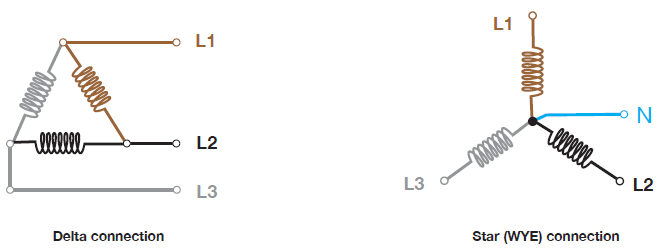Figure 20 - Three-phase delta and star (WYE) configurations

#### Blondel’s Theorem

When discussing power measurements with wattmeters, Blondel’s Theorem is often referenced when determining the correct  method of connecting the wattmeters and how many are required for the most accurate measurement. The Theorem states that the power provided to a system of N conductors is equal to the algebraic sum of the power measured by N wattmeters. Additionally, if a common point is located on one of the conductors, that conductor’s meter can be removed and only N-1 meters are required.

#### Three-phase Star Connection (3P4W)

Measurement is relatively simple if the measurement object is a three-phase 4-wire system. As shown in the diagram below, three-phase 4-wire involves connecting wattmeters to each phase based on a neutral conductor. Obtain power for each phase by measuring voltage (phase voltage) and current (phase current) for each phase with different wattmeters. Totaling this will give the three-phase AC power value. Measuring three-phase 4-wire power requires three wattmeters.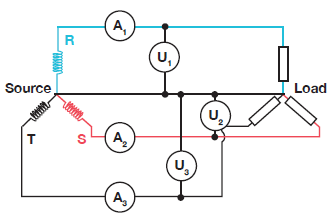Figure 21 - Three-phase star connection (3P4W)

Apparent power, active power, and reactive power for three-phase power is the total of each phase.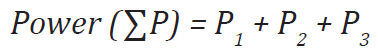#### Three-phase Delta Two Wattmeter (3P3W)

Measurement with the three-phase 3-wire system is a bit more complicated because the neutral conductor that was used as the base for the three-phase 4-wire system is absent and phase voltage cannot be measured. Measurement under the three-phase 3-wire system involves obtaining the three-phase AC power value using a method called the 2-wattmeter method.

By applying Blondel's theorem and using the two-wattmeter method, we can obtain three-phase AC power values. A connection diagram for the two-wattmeter method and a vector map are shown on below.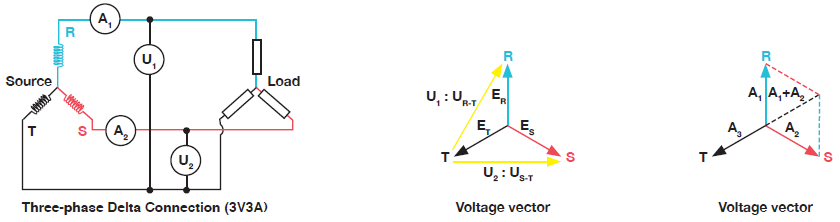The derivation of Blondel’s Theorem is below.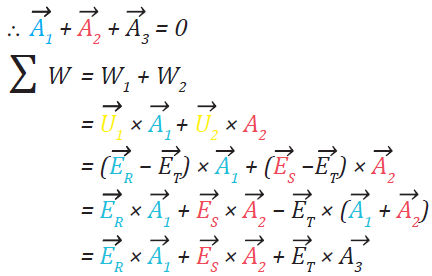The calculation above shows that we can obtain three-phase AC power values from two-line power values and two-phase current values. Since this method requires monitoring only two current and two voltages instead of three, installation and wiring configuration are simplified. It can also measure power accurately on a balanced or an unbalanced system. Its flexibility and low-cost installation make it a good fit for production testing in which only the power or a few other parameters need measurement.

In other words, for three-phase power measuring, power can be obtained by measuring power for each phase and calculating the total. For the two wattmeter method, the equation is shown below.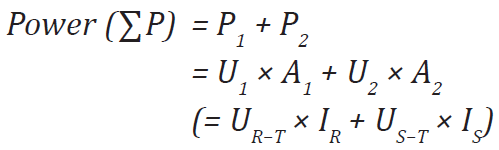#### Three-phase Delta Connection (3V3A)

There is one more measurement method under the three-phase 3-wire system: three-voltage three-current measurement (3V3A). Like the two-wattmeter method, this method measures T phase current and line voltage between R and S. The following is a connection diagram.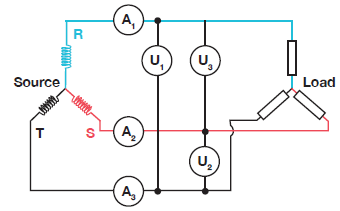Figure 22 - Three-phase delta connection (3V3A)

As the three-voltage three-current method (3V3A) measures T phase current, it allows for seeing the current balance among the phases that was not possible using the two-wattmeter method. For engineering and research and development work, the three-phase

three-wire with three-wattmeter method is best as it provides additional information that can be used to balance loading and determine true power factor. This method uses all three voltages and all three currents. All three voltages are measured (R to T, S to T, R to S).

#### Vector Display of Three-phase AC Measurements

We will use a three-phase Y “star” system to illustrate the concept of a three phase vector display. In a star system, the voltages and currents of each phase are offset by 120°. The neutral point of a Y system is at the center where all voltages and currents theoretically sum to zero.

When making measurements on a star system where a physical neutral wire is present; voltages will be measured with respect to this neutral point, this is called “phase voltage”. When making measurements on a star system where a physical neutral wire is not present; the voltages will be measured with respect to each other, this is called “line voltage” or “delta wiring”. Delta wiring forms an equilateral triangle with 60 degree spacing between voltages, versus star wiring where voltages are paced by 120 degrees. Themagnitude of line voltages measure higher than the phase voltages by a factor of √3. Currents in a star system are always measured in series with respect to the neutral point, with the angular measurement with respect to voltage vectors denoted by Φ. Figure 23 illustrates the relationships of a delta-wired vs a star-wired measurement of voltages with a vector diagram.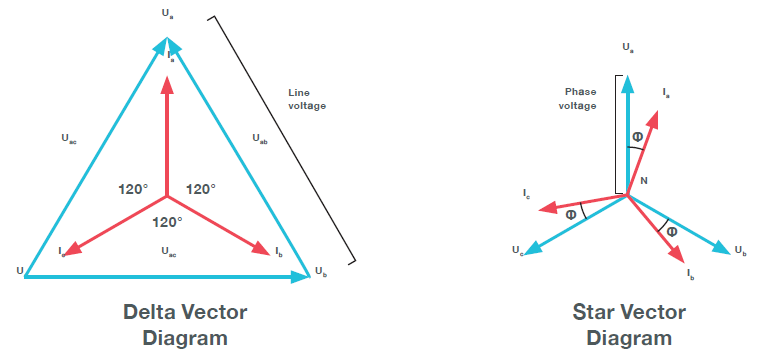Figure 23 - Vector diagram of three-phase delta and star measurements.

#### Three-phase Power Factor Measurement

Total power factor for a 3-phase circuit is determined by summing the total watt divided by the total VA measurement.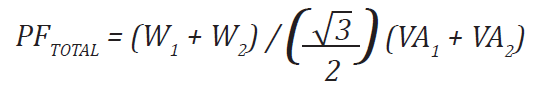Using the two-wattmeter method, the sum of the total watt (W1 + W2) is divided by the VA measurements. However, if the load is unbalanced (the phase currents are different), this could introduce an error in calculating the power factor because only two VA measurements are used in the calculation. The two VAs are averaged because it's assumed they're equal; however, if they're not, a faulty result is obtained. Therefore, it's best to use the three-wattmeter method for unbalanced loads because it will provide a correct power factor calculation for either balanced or unbalanced loads.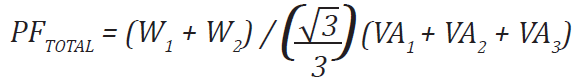With the three-wattmeter method, all three VA measurements are used in the above power factor calculation.

### Harmonics

Harmonics refer to all sine waves whose frequency is an integer multiple of the fundamental wave (normally a 50 Hz or 60 Hz sinusoidal power line signal, or between 0 and 2kHz for rotating machines). Harmonics are a distortion of the normal electrical current waveforms generally transmitted by nonlinear loads. Unlike linear loads where the current drawn is proportional to and follows the waveform of the input voltage, non-linear loads like variable speed motors draw current in short abrupt pulses. When the fundamental wave and subsequent harmonic components are combined, waveforms become distorted, and interference occurs.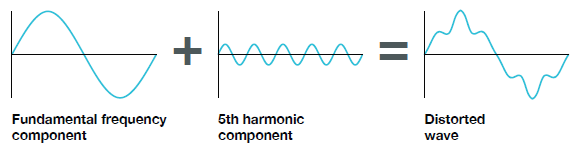Figure 24 - Distorted waveforms consist of multiple harmonic components

Harmonics must be controlled as they can cause abnormal noise, vibration, heat, or improper operation in devices and shorten their life. Domestic and international standards such as IEC61000-3 exist for the purpose of harmonic control. Engineers therefore need to detect harmonics and assess their effects on components, systems, and subsystems within an application. The size and phase difference should be measured for not only the fundamental frequency but also for each higher frequency component. Highly accurate power analyzers can measure harmonics upwards of the 500th order.

For rotating machines, the fundamental amplitudes are the only components that effectively contribute to the rotation of the axle all other harmonic components result in losses in the form of heat and vibration.

#### Measuring Harmonics

Using the harmonic measurement mode, size and phase difference can be measured for each fundamental frequency as well as harmonics for each degree included in current, voltage, and power. In the case of a fundamental frequency (primary component) of 50 Hz, for example, the third component is 150 Hz, the fifth component is 250 Hz, and so on, and measurement up to the 500th component at 2.5 kHz is possible.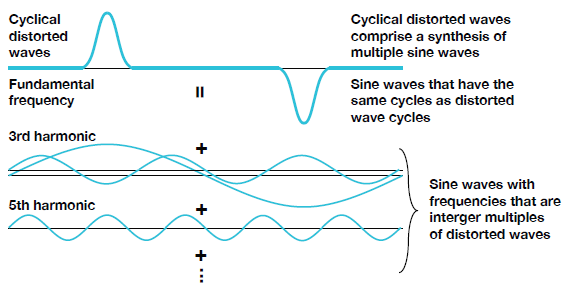Figure 25 - Odd harmonic components sum into a distorted waveform

For the display of harmonic measurement results, a power analyzer can show the size of each degree as shown in Figure 26 below, or show things such as size, content ratio, and phase in a list.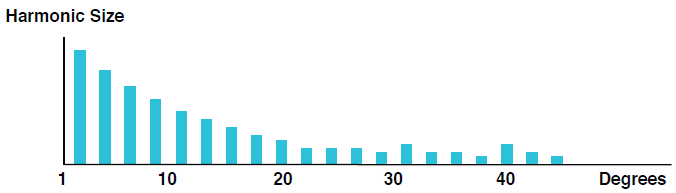Figure 26 - Bar chart showing harmonic energy versus order

### Conclusion

Many items need to be considered when measuring power, including input power, inverter efficiency, efficiency, harmonics, and power factor. These measurements involve complicated equations, which is why most companies employ a power analyzer to generate results automatically.

A precision high-frequency power analyzer is an important tool for measuring both mechanical and electrical power. Its analysis features and readings can help improve operations and even extend the life of a motor. Selecting the right analyzer and implementing it correctly does require knowledge; however, if employed correctly the power analyzer data will provide accurate and highly valuable data.

### Related Products & Solutions

#### Data Acquisition (DAQ)

Yokogawa data acquisition systems give you the most flexibility and power to measure, display, store, and even actuate any number of physical or electrical phenomena.

#### Oscilloscopes

• Digital oscilloscopes
• High-speed sampling
• Range of bandwidths for electronic device design and development
• Advantages of oscilloscope and multi-channel data recorder

#### Power Analyzers and Power Meters

Yokogawa, the world's largest manufacturer of energy and power analyzers and meters, provides a broad choice of digital power analyzers to satisfy all requirements.

#### ScopeCorders

• Flexible, high-performance Yokogawa ScopeCorder
• Modular platform combines mixed signal oscilloscope and portable data acquisition recorder
• Captures high-speed transients and low-speed trends

Top